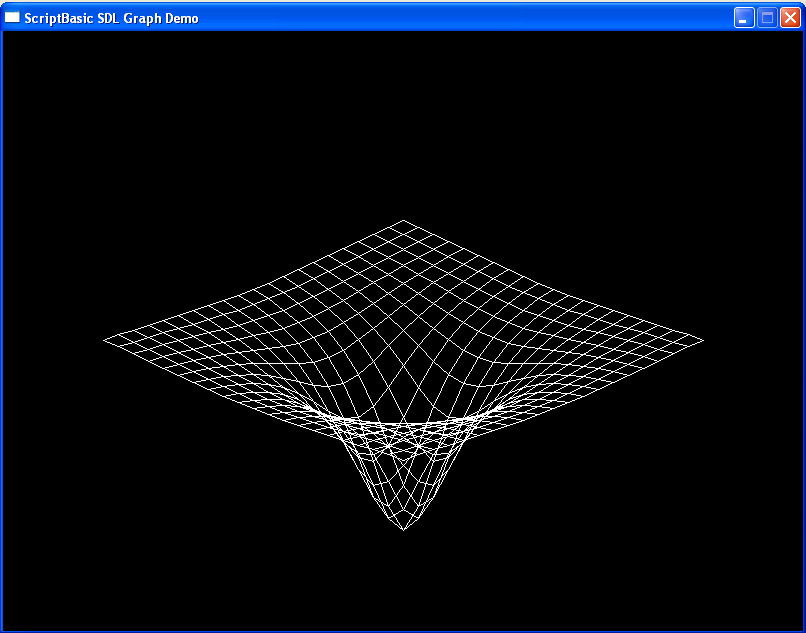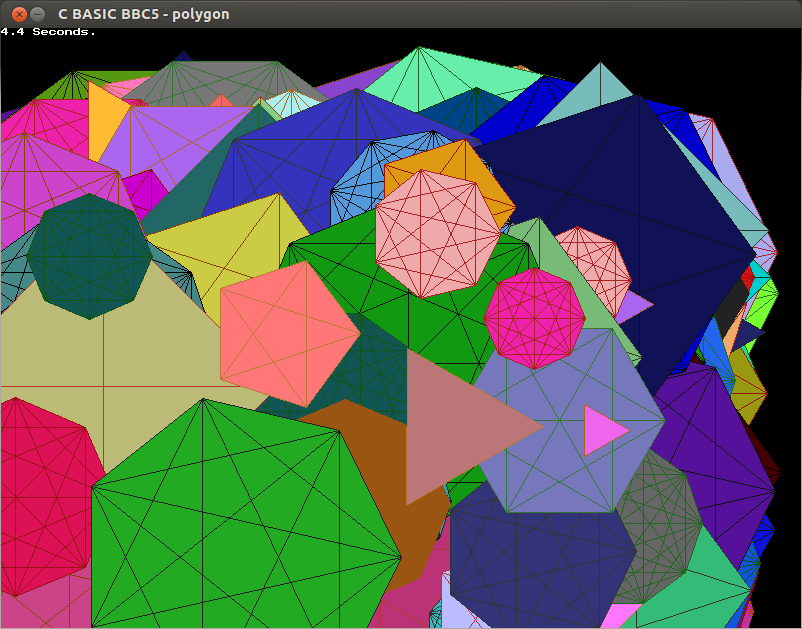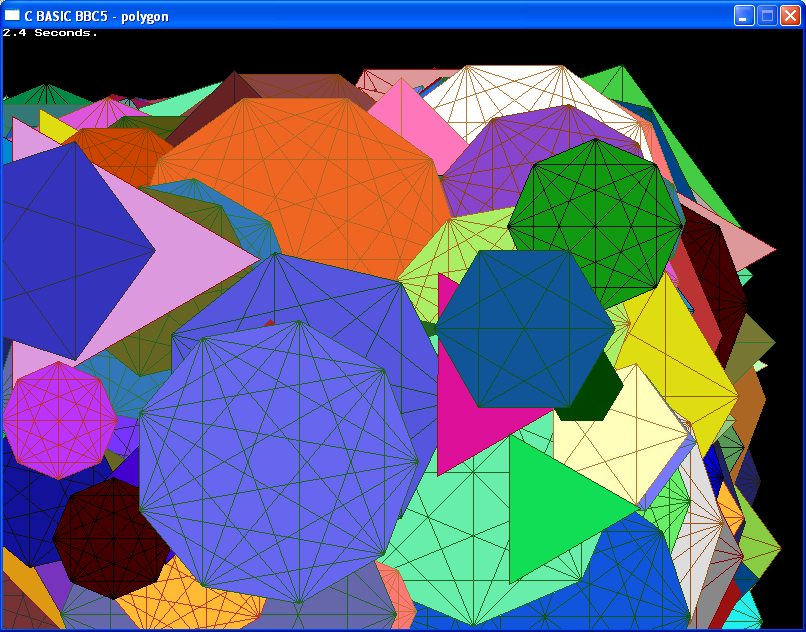###Author Topic: C BASIC - BBC Graphics Library  (Read 4706 times)

####John##### C BASIC - BBC Graphics Library
« on: February 08, 2014, 12:53:08 AM »
I have posted on Bitbucket the C BASIC version of the Brandy BASIC V SDL library converted to a shared object. This version no longer requires the Brandy BASIC V distribution to build the library. This version is basically a conversion and cleanup with no new functionality from the last C source build. The goal with this release is to make sure I didn't break anything in the conversion. The BaCon guys are doing a great job of pushing this forward and I hope this works out as a popular library for them.

If you want to see the libbbc.c in it's naked form, you can view it here.

.25 cents / peep

Note: The C BASIC version is the only copy being maintained on Bitbucket. If you wish to create your own native C version of the library, do the following and start at the end of the redirected file and work your way up to the first function in the library. Add the header and includes (- cbasic.h) and that's all there is to it.

gcc -E -I/usr/include/SDL libbbc.c > libbbc.expanded

Update

I updated the files on Bitbucket to fix a typo and did more housekeeping with the code. So far everything I tested is working fine with the new code base.

I've attached a Windows 32 bit DLL and the source if anyone would like to try it on that platform.
« Last Edit: February 08, 2014, 10:21:12 PM by John »

####John##### Re: C BASIC - BBC Graphics Library
« Reply #1 on: February 09, 2014, 02:42:32 AM »
I thought I would try doing the graphdemo using C BASIC.  I have created a work in progress version of the BBC BASIC graphics library which I'm calling libbbc5.c. (original libbbc.c is still on Bitbucket) The goal is a generic BBC BASIC graphics plug-in any language can bind to without having any SDL knowledge per say. It will handle the keyboard / mouse events along with a few BBC BASIC specific functions (BBC_RND, BBC_TIME, ...) to help port BBC programs to the language of your choice. I plan to convert the existing examples to C BASIC and post them on the Bitbucket site.

Update: I added BBC_WAITKEY, BBC_GETKEY and BBC_RND to the libbbc5 library. The most current version is on Bitbucket if you're following along.Code: [Select]
`// Graph Demo#include <SDL.h>#include "libbbc5.h"#include "cbasic.h"MAINBEGIN_FUNCTION  DIM AS long xlow, xhigh, ylow, yhigh, depth, xscale, yscale, c, x, y;   BBC_OPEN();  SDL_WM_SetCaption("C BASIC BBC graphdemo", 0);  BBC_MODE(31);  BBC_ORIGIN(800, 600);  xlow = -10;  xhigh = 10;  ylow = -10;  yhigh = 10;  depth = 10;  xscale = 30;  yscale = 12;  c = -4000;  x = 0;  y = 0;    DEF_FOR (x = xlow TO x <= xhigh STEP x += 1)  BEGIN_FOR     BBC_MOVE(xscale*(x+ylow), yscale*(ylow-x)+c/(x*x+ylow*ylow+depth));    DEF_FOR (y = ylow TO y <= yhigh STEP y += 1)    BEGIN_FOR      BBC_DRAW(xscale*(x+y), yscale*(y-x)+c/(x*x+y*y+depth));    NEXT  NEXT  DEF_FOR (y = ylow TO y <= yhigh STEP y += 1)  BEGIN_FOR    BBC_MOVE(xscale*(xlow+y), yscale*(y-xlow)+c/(xlow*xlow+y*y+depth));    DEF_FOR (x = xlow TO x <= xhigh STEP x += 1)    BEGIN_FOR      BBC_DRAW(xscale*(x+y), yscale*(y-x)+c/(x*x+y*y+depth));    NEXT  NEXT// BBC_WAITKEY();   DEF_WHILE (BBC_GETKEY() != SDLK_ESCAPE)   BEGIN_WHILE   WEND  BBC_CLOSE();  RETURN_FUNCTION(0);END_FUNCTION`

This is the libbbc.dll version running under Windows with ScriptBasic and DLLC.Code: [Select]
`' Graph DemoINCLUDE "dllcinc.sb"bbc = dllfile("libbbc.dll")sdl = dllfile("sdl.dll")BBC_OPEN = dllproc(bbc,"init_screen i = ()")BBC_MODE = dllproc(bbc,"emulate_mode (i mode)")BBC_ORIGIN = dllproc(bbc,"emulate_origin (i x, i y)")BBC_DRAW = dllproc(bbc,"emulate_draw (i x, i y)")BBC_MOVE = dllproc(bbc,"emulate_move (i x, i y)")BBC_CLOSE = dllproc(bbc,"end_screen ()")SDL_WINTITLE = dllproc(sdl,"SDL_WM_SetCaption (z title, z icon)")SDL_WAIT = dllproc(sdl,"SDL_Delay (i millsecs)")dllcall BBC_OPENdllcall SDL_WINTITLE, "ScriptBasic SDL Graph Demo", "ScriptBasic"dllcall BBC_MODE, 31dllcall BBC_ORIGIN, 800, 600xlow = -10xhigh = 10ylow = -10yhigh = 10depth = 10xscale = 30yscale = 12c = -4000FOR x = xlow TO xhigh  dllcall BBC_MOVE, xscale*(x+ylow), yscale*(ylow-x)+c/(x*x+ylow*ylow+depth)  FOR y = ylow TO yhigh    dllcall BBC_DRAW, xscale*(x+y), yscale*(y-x)+c/(x*x+y*y+depth)  NEXTNEXTFOR y = ylow TO yhigh  dllcall BBC_MOVE, xscale*(xlow+y), yscale*(y-xlow)+c/(xlow*xlow+y*y+depth)  FOR x = xlow TO xhigh    dllcall BBC_DRAW, xscale*(x+y), yscale*(y-x)+c/(x*x+y*y+depth)  NEXTNEXTdllcall SDL_WAIT, 5000dllcall BBC_CLOSE`
« Last Edit: February 09, 2014, 11:15:40 PM by John »

####John##### Re: C BASIC - BBC Graphics Library
« Reply #2 on: February 10, 2014, 08:30:20 PM »
Here is the polygon BBC BASIC V example done in C BASIC. I wanted to make sure the BBC_RND function was working in the library. I also added Charles Pegge's BBC_SHIFT and BBC_ROTATE functions to the library. (see BBC_SHIFT used to replace the C << left shift operator)

Note: All further development is being done on the libbbc5.c code set and the original libbbc.c files will be removed from Bitbucket soon.Code: [Select]
`// C BASIC Polygon#include <stdio.h>#include <math.h>#include <SDL.h>#include "libbbc5.h"#include "cbasic.h"#define PI 3.14159265358979323846MAINBEGIN_FUNCTION  DIM AS float64 angle, radius, x, y;  DIM AS int32 c, t, d, side, sides, xorigin, yorigin, i, l;  DIM AS Uint32 t1, t2, t3;  DIM AS char strbuff;  BBC_OPEN();  BBC_MODE(32);  t1 = SDL_GetTicks();  SDL_WM_SetCaption("C BASIC BBC5 - polygon", 0);  BBC_VDU(26);  DEF_FOR (i = 1 TO i <= 1000 STEP INCR i)  BEGIN_FOR    xorigin = BBC_RND(1250);    yorigin = BBC_RND(840);    radius = BBC_RND(300) + 50;    BBC_ORIGIN(xorigin, yorigin);    sides = BBC_RND(8) + 2;    BBC_MOVE(radius, 0);    BBC_MOVE(10, 10);    c = BBC_RND(64) - 1;    t = BBC_SHIFT(BBC_RND(4) - 1, 6, 0);    BBC_GCOL(0, c, t);    DEF_FOR (side = 1 TO side <= sides STEP INCR side)    BEGIN_FOR      angle = (side -1) * 2 * PI / sides;      x[side] = radius * cos(angle);      y[side] = radius * sin(angle);      BBC_MOVE(0, 0);      BBC_PLOT(85, x[side], y[side]);    NEXT    BBC_MOVE(0, 0);    BBC_PLOT(85, radius, 0);    DO      d = BBC_RND(64) - 1;    WHILE ((d & 63) != (c & 6));    BBC_GCOL(0, d, t);    DEF_FOR (side = 1 TO side <= sides STEP INCR side)    BEGIN_FOR      DEF_FOR (l = side TO l <= sides STEP INCR l)      BEGIN_FOR        BBC_MOVE(x[side], y[side]);        BBC_DRAW(x[l], y[l]);      NEXT    NEXT  NEXT  t2 = SDL_GetTicks();  t3 = (t2 - t1) / 1000;  sprintf(strbuff, "%d.4 Seconds.", t3);  BBC_OFF();  BBC_VDUSTR(strbuff, 0);  BBC_WAITKEY();  BBC_CLOSE();  RETURN_FUNCTION(0);END_FUNCTION`« Last Edit: February 11, 2014, 02:10:21 AM by John »

####John##### Re: C BASIC - BBC Graphics Library
« Reply #3 on: February 11, 2014, 02:12:09 PM »
I compiled the latest libbbc5.dll for Windows 32 bit along with the C BASIC version of the BBC polygon example. Everything is attached if you would like to give it a try yourself. (SDL 1.2 installed is a requirement)Code: [Select]
`// C BASIC Polygon#include <stdio.h>#include <math.h>#include <SDL.h>#include "libbbc5.h"#include "cbasic.h"#define PI 3.14159265358979323846MAINBEGIN_FUNCTION  DIM AS float64 angle, radius, x, y;  DIM AS int32 c, t, d, side, sides, xorigin, yorigin, i, l;  DIM AS Uint32 t1, t2, t3;  DIM AS char strbuff;  BBC_OPEN();  BBC_MODE(32);  t1 = SDL_GetTicks();  SDL_WM_SetCaption("C BASIC BBC5 - polygon", 0);  BBC_VDU(26);  DEF_FOR (i = 1 TO i <= 1000 STEP INCR i)  BEGIN_FOR    xorigin = BBC_RND(1250);    yorigin = BBC_RND(840);    radius = BBC_RND(300) + 50;    BBC_ORIGIN(xorigin, yorigin);    sides = BBC_RND(8) + 2;    BBC_MOVE(radius, 0);    BBC_MOVE(10, 10);    c = BBC_RND(64) - 1;    t = BBC_SHIFT(BBC_RND(4) - 1, 6, 0);    BBC_GCOL(0, c, t);    DEF_FOR (side = 1 TO side <= sides STEP INCR side)    BEGIN_FOR      angle = (side -1) * 2 * PI / sides;      x[side] = radius * cos(angle);      y[side] = radius * sin(angle);      BBC_MOVE(0, 0);      BBC_PLOT(85, x[side], y[side]);    NEXT    BBC_MOVE(0, 0);    BBC_PLOT(85, radius, 0);    DO      d = BBC_RND(64) - 1;    WHILE ((d & 63) != (c & 6));    BBC_GCOL(0, d, t);    DEF_FOR (side = 1 TO side <= sides STEP INCR side)    BEGIN_FOR      DEF_FOR (l = side TO l <= sides STEP INCR l)      BEGIN_FOR        BBC_MOVE(x[side], y[side]);        BBC_DRAW(x[l], y[l]);      NEXT    NEXT  NEXT  t2 = SDL_GetTicks();  t3 = (t2 - t1) / 1000;  sprintf(strbuff, "%d.4 Seconds.", t3);  BBC_OFF();  BBC_VDUSTR(strbuff, 0);  DEF_WHILE (BBC_GETKEY() != SDLK_ESCAPE)   BEGIN_WHILE  WEND//  BBC_WAITKEY();  BBC_CLOSE();  RETURN_FUNCTION(0);END_FUNCTION`
Code: [Select]
`F:\libbbc>gcc polygon.c -o polygon.exe -I C:/MinGW32/include/SDL -D_GNU_SOURCE=1 -D_REENTRANT -DHAVE_OPENGL -lmingw32 lSDLmain -lSDL -mwindows libbbc5.dll -lm`

####John##### Re: C BASIC - BBC Graphics Library
« Reply #4 on: February 11, 2014, 05:47:08 PM »
Here is the Android C BASIC version of the BBC5 polygon example. The same C BASIC libbbc5 library and example code ran untouched on 32 and 64 bit systems including ARM. I don't own any Apple gear but SDL is suppose to work on that platform as well.« Last Edit: February 11, 2014, 07:02:01 PM by John »

####John##### Re: C BASIC - BBC Graphics Library
« Reply #5 on: February 12, 2014, 03:38:32 PM »
I added BBC_MOUSE based on vovchik's routine.

Code: [Select]
`#include <stdio.h>#include <math.h>#include <SDL.h>#include "libbbc5.h"#include "cbasic.h"MAINBEGIN_FUNCTION  DIM AS int m, i;  BBC_OPEN();  DEF_FOR (i = 1 TO i <= 3 STEP INCR i)  BEGIN_FOR    m = BBC_MOUSE(2);    PRINT ("Button: = %i\n", m);    m = BBC_MOUSE(0);    PRINT ("X: = %i\n", m);    m = BBC_MOUSE(1);    PRINT ("Y: = %i\n", m);  NEXT  BBC_WAITKEY();  BBC_CLOSE();  RETURN_FUNCTION(0);END_FUNCTION  `
jrs@laptop:~/libbbc\$ ./mouse
Button: = 1
X: = 426
Y: = 8
Button: = 2
X: = 461
Y: = 164
Button: = 3
X: = 173
Y: = 362
jrs@laptop:~/libbbc\$

Update

I think I have the BBC_MOUSE and BBC_GETKEY working in both polling or wait modes for events.

BBC_MOUSE(0) - Waits for mouse movement and returns X position.
BBC_MOUSE(1) - Waits for mouse movement and returns Y position.
BBC_MOUSE(2) - Checks for a mouse button press event and returns.
BBC_MOUSE(3) - Waits for a mouse button to be pressed then returns.

BBC_GETKEY(0) - Polls for a key press event and returns.
BBC_GETKEY(1) - Waits for a key to be pressed then returns.

This really needs some testing and a good example program.
« Last Edit: February 12, 2014, 09:47:29 PM by John »

####John##### Re: C BASIC - BBC Graphics Library
« Reply #6 on: February 13, 2014, 10:03:25 AM »
I'm beginning to realize the limitations of C BASIC not having memory management (string) support. I hope with this BBC BASIC graphic library project it renewed the interest in C BASIC and maybe moving it forward beyond just an illusion that takes the C jitters away.

The libbbc5 library as a standalone BBC BASIC graphics binding seems to be working and I hope others will improve on it. I'm just frustrating myself taking it any further without string and memory management support in C BASIC. With that said, I'm going to generate a subset of the ScriptBasic SDL extension module that only includes the BBC functionality. I'll release a Linux and Windows version of the bbc.so/bbc.dll extension modules for ScriptBasic users.

Thanks to all who contributed to the libbbc5 project.

« Last Edit: February 13, 2014, 01:44:17 PM by John »